Courses

# Construction Management Civil Engineering (CE) Notes | EduRev

## Civil Engineering (CE) : Construction Management Civil Engineering (CE) Notes | EduRev

The document Construction Management Civil Engineering (CE) Notes | EduRev is a part of the Civil Engineering (CE) Course Topic wise GATE Past Year Papers for Civil Engineering.
All you need of Civil Engineering (CE) at this link: Civil Engineering (CE)

Question 1: The network of a small construction project awarded to a contractors is shown in the following figure. The normal duration, crash duration, normal cost and crash cost of all the activities are shown in the table. The indirect cost incurred by the contractor in I NR 5000 per day.    [2019 : 2 Marks, Set-1]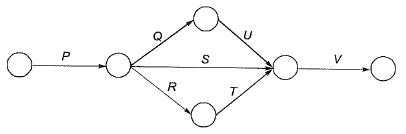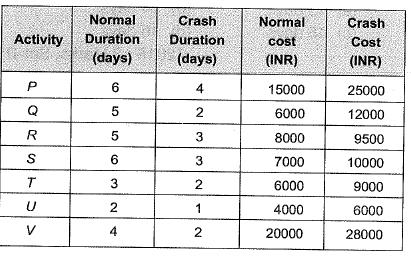If the project is target for completion in 16 days, the total cost (in INR) to be incurred by the contractor would be_______.

Solution: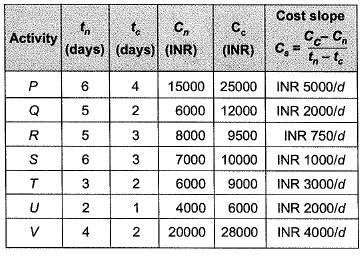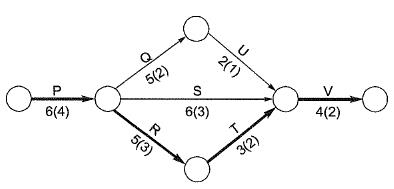Considering normal durations, the total cost of the project
TC = DC + IC
= INR 66000 + INR 5000 x 18
= INR 156000
Stage-I: Crash activity R by 1 day
∴ Project becomes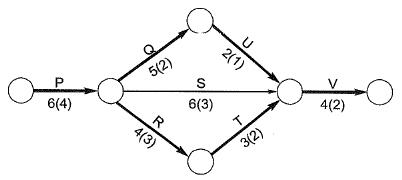TC = (INR 6600 + INR 750) + INR 5000 x 17
= 151750
Stage-II: Crash activity Q&R (or U&R) by 1 day
∴ Project becomes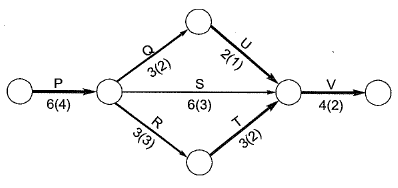TC = (INR 66750 + INR 2000 + INR 750) + (INR 5000 x 16)
= 149500

Question 2: For a construction project, the mean and standard deviation of the completion time are 200 days and 6.1 days, respectively. Assume normal distribution and use the value of standard normal deviate, z = 1.64 for the 95% confidence level. The maximum time required (in days) for the completion of the project would be _____.     [2017 :1 Mark, Set-II]
Solution: Expected completion time of project,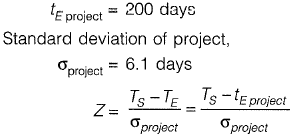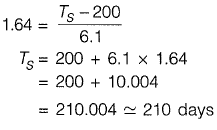Question 3: Given that the scope of the construction work is well-defined with all its drawings, specifications, quantities and estimates, which one of the following types of contract would be most preferred?    [2017 : 1 Mark, Set-II]
(a) EPC contract
(b) Percentage rate contract
(c) Item rate contract
(d) Lump sum contract
Answer: (c)
Solution: Percentage rate Contract: In this form of contract, the displacemente draws up 'Item rate tender' i.e., bill of quantities with-rate, amount and total amount. The contractors are required to offer to carry out the work as per the rates shown in the specifi price schedule or some percenrage above or some percentage below the rates indicated in the shedule of work attached with the tender.
Item rate contract: In this a contract under takes the execution of work on an item rate basis. He is required to quote rate for individual item of work o the basis of shedule of quantities i.e., BOQ furnished by the department.
Lumpsum concract: In this type, a contractor is required to quote a fixed sum for execution of work complete in all respect in the stipulated time according to the drawing, design and specification supportd to him with the tender. In item rate contract, contractor only fill its rate against given item and its quantity and contract does not change the item make, drawing, specification during construction without consent of owner/department.
Item rate contract is also called “unit price contract” where contractor bids cost per unit of each item. These works are carried out in accordance with contract documents. The contractor has to perform the quantities of the work actually required in the field at his quoted unit prices, whether they are less or greater than “owner/department” estimates.

Question 4: The activity details of a project are given below:    [2017 : 2 Marks, Set-I]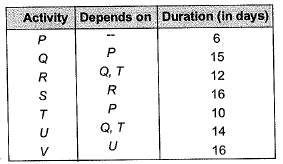The estimated minimum time (in days) for the completion of the project will be _____ .
Solution: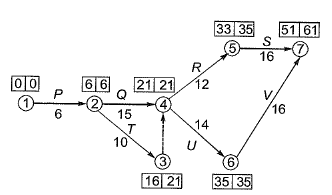Note: Path of longest duration is defined as the critical path as the activities lying in this path are called critical activities. Any delay in these activity will lead to delay in overall project. The project time given by critical path. Hence the time taken by the critical path is the shortest possible time to finish the project.
Critical Path → P - Q - U - V
Tcp = 6 + 15 + 14 + 16 = 51 days

Question 5: The activity-on-arrow network of activities for a construction project is shown in the figure. The durations (expressed in days) of the activities are mentioned below the arrows.    [2016 : 2 Marks, Set-II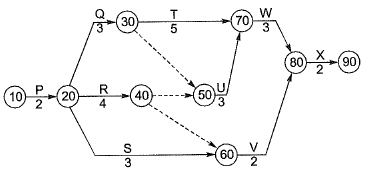The critical duration for this construction project is
(a) 13 days
(b) 14 days
(c) 15 days
(d) 16 days
Answer: (c)
Solution: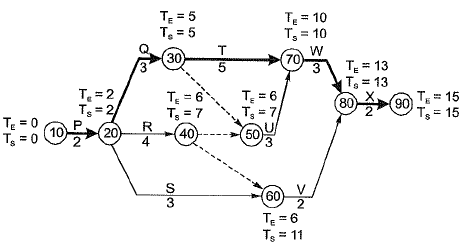The critical duration is 15 days which is observed along the path P-Q-T-W-X which is the critical path of the project.
Note: Critical path is the one which consumes maximum amount of time.

Question 6: The Optimistic Time (O), Most likely Time (M) and Pessimistic Time (P) (in days) of the activities in the critical path are given below in the format O-M-P.   [2016 : 2 Marks, Set-I]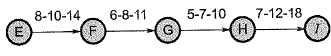The expected completion time (in days) of the project is __________
Solution: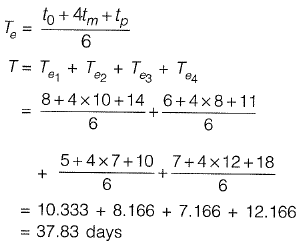Question 7: A construction project consists of twelve activities The estimated duration (in days) required to complete each of the activities along with the corresponding network diagram is shown below.    [2016 : 1 Mark, Set-II]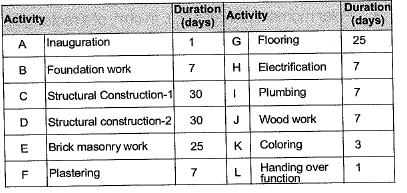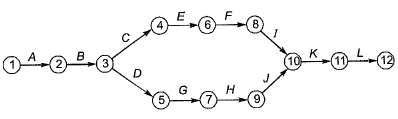Total floats (in days) for the activities 5-7 and 11-12 for the project are, respectively,
(a) 25 and 1
(b) 1 and 1
(c) 0 and 0
(d) 81 and 0
Answer: (c)
Solution: Total float can be determined once the activity times i.e. EST, EFT, LST and LFT are known.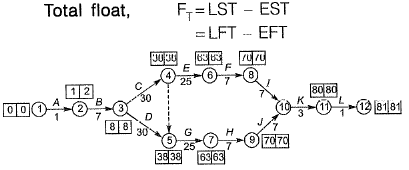For activity 5-7,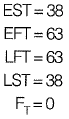For activity 11-12,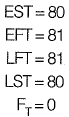Note: It can be seen directly that since the slack of all events are zero, there is not margin left for the occurence of events and therefore.
Maximum available line = Time required for completion of activity
∴ FT for all activities is zero.

Offer running on EduRev: Apply code STAYHOME200 to get INR 200 off on our premium plan EduRev Infinity!

69 docs

,

,

,

,

,

,

,

,

,

,

,

,

,

,

,

,

,

,

,

,

,

;